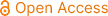### Applied and Computational Mathematics

Archive
• 2023, Volume 12

• 2022, Volume 11

• 2021, Volume 10

• 2020, Volume 9

• 2019, Volume 8

• 2018, Volume 7

• 2017, Volume 6

• 2016, Volume 5

• 2015, Volume 4

• 2014, Volume 3

• 2013, Volume 2

• 2012, Volume 1

Submit a Manuscript

Publishing with us to make your research visible to the widest possible audience.Propose a Special Issue

Building a community of authors and readers to discuss the latest research and develop new ideas.### A q-Operational Equation for Carlitz’s q-Operators with Some Applications

Rogers–Szegö polynomials are the basis in the Scheme of basic hypergeometric orthogonal polynomials. By solving a q-operational equation with formal power series, Liu introduced a new q-exponential operational identity and developed a systematic method to prove the identities involving the Rogers–Szegö polynomials. In this paper, motivated by Carlitz’s q-operators and Liu’s q-operational equation, we construct an q-operational equation for Carlitz’s q-operators and give some applications to some generating functions for Rogers–Szegö polynomials and Hahn polynomials, which generalize the method of exponential operator decomposition introduced by Cao and provide a new proof of results of Carlitz and Saad et al.. We chose Mehler’s formula, q-Nielsen’s formula for Rogers–Szegö polynomials and Mehler’s formula for Hahn polynomials as examples to show that the q-series theory can be applied, which takes us quickly the results. One of the main characteristics of this method is that it provides an effective approach to calculate generating functions for some q-polynomials. This method also brings a new research perspective to problems of the sum and integration of q-polynomials.

q-Operational Equation, Carlitz’s q-operators, Rogers–Szegö Polynomials, Hahn Polynomials, Generating Function

Jian Cao, Cheng Zhang, Sama Arjika. (2023). A q-Operational Equation for Carlitz’s q-Operators with Some Applications. Applied and Computational Mathematics, 12(4), 92-108. https://doi.org/10.11648/j.acm.20231204.12